﻿ Delayed choice quantum eraser experiment real ?

# Delayed choice experiment is a real thing ?

## Wheeler's delayed choice experiment can be explained by classical waves.

### Can we really change ( choose ) the past events ??

In fact, the illusory quantum photon particle instead of the real classical light wave causes fictional delayed choice quantum eraser misinterpretation.

Wheeler's delayed choice experiment is a thought experiment proposed by John Wheeler in 1978, and is confirmed experimentally later.

Surprisingly, in this experiment, we can choose (change) the photon's past state from the future !
This means we can control the past events from the future.
But in this real world, is it really possible ??
Here we try to explain the "tricks" of these mysterious phenomena.

## Mach-Zehnder interferometer shows wave-particle of a photon ?

(Fig.1) "Particle" nature of a photon is observed ?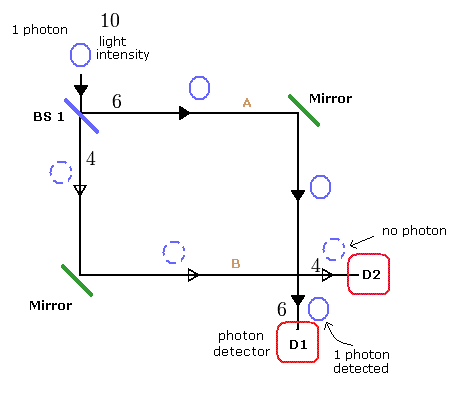Fig.1 is a Mach-Zehnder interferometer.
In Fig.1, a single photon is split at the half beam splitter 1 ( BS 1 ).
(In the half beam splitter, almost half of the light is reflected, and the remaing half is transmitted. )
Of course, according to the quantum mechanical interpretation, a single photon can NOT be divided by the splitter.

## A single photon is through ONLY one of two paths ?

So the single photon exists in only one of the two paths after the beam splitter according to the quantum mechanics.
When we detect a photon at the detectors D1 and D2, we can detect the single photon at only one of these two detectors at the same time. ( D1 or D2 )
(We can NOT detect the photon at D1 and D2 at the same time.)

This experimental result shows that the photon is a particle.
In Fig.1 when we detect the single photon at D1, it shows the photon has passed the A route.
( When we detect at D2, it has passed B route. )
So we can get the photon which-path information in Fig.1, and in this case the photon shows "particle" nature.

## A single photon, but interference from two paths is observed !

(Fig.2) "Wave" nature of a photon is observed ?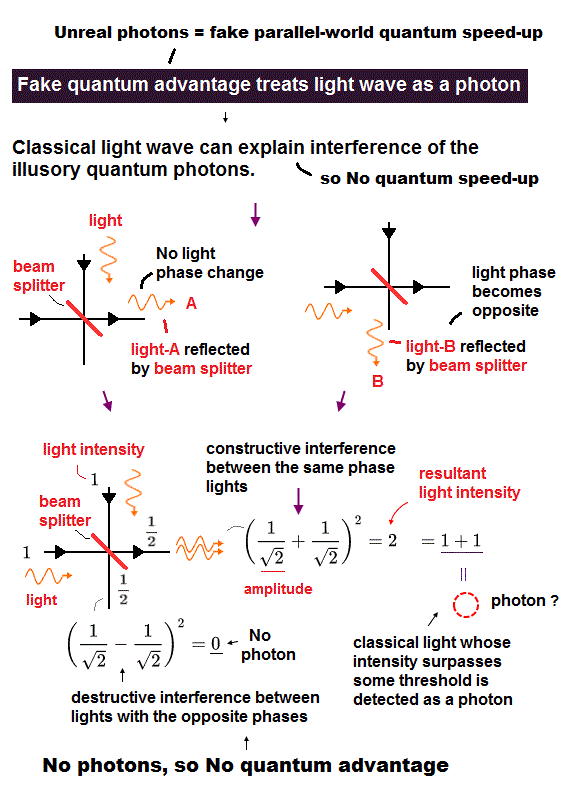In Fig.2, we insert the half beam splitter 2 (BS 2), too.
We suppose the beam splitter 2 ( BS 2 ) reverses the wave phase of only one reflection (downward), as shown in Fig.2.
( The transmitted light doesn't change. )

As a result, we always detect the single photon only at D2 detector by the interference from the A and B paths.
And at D1 detector, the electromagnetic waves from the A and B paths cancel each other. So we can not detect the photon at D1.
This means the photon shows "wave" nature by inserting the beam splitter 2 !

## Delayed choice = we can change past events !?

This is a very mysterious thing.
According to Fig.1, after the photon has passed the beam splitter 1 ( BS 1 ), the photon becomes "particle".
But if we insert the beam splitter 2 after the photon has passed BS 1, the photon particle changes to the waves !
This means that we can change the past events ( particle or wave ) from the future ! (= delayed choice )
But in this real world, is it really possible ?

## Wave or particle can be determined from the future !?

(Fig.3) Can we change (choose) the past event ( particle or wave ) ?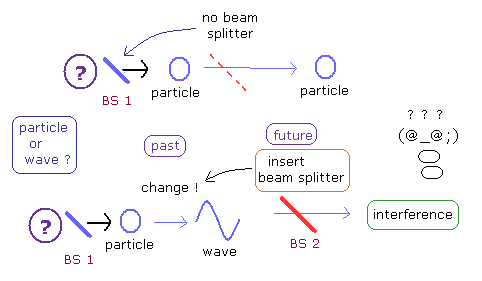## Delayed choice experiment = classical electromagnetic waves

Actually, we can explain these mysterious phenomena of the delayed choice experiment ONLY by the classical electromagntic ( E-M ) waves .
Here we show this mechanism.

As shown on this page, We suppose the photon detector can detect it when the light intensity is 6 as a single photon.
( So when its light intensity is less than 6, it can not be recognized at the detector as a photon, even if the electromagnetic wave exists. )

## Classical wave + "detection threshold" is the trick of delayed choice.

For example, the first light intensity is 10, then it is split by the beam splitter ( BS1 ) into 6 + 4 waves.
( 10 is detected as one photon, not 2 photons (=12). )
The 4 side is not detected, only 6 side ( D1 or D2 ) is detected as a photon due to the detection threshold.
( If the E-M waves is split into 5 + 5, no photons can be detected. This case is not recognized at all, so it can be ignored. )
So we can explain the "particle" nature of the photon of Fig.1 by the classical electromagnetic waves.

When we insert another half beam splitter 2 ( BS 2 ) as shown in Fig.2, "6" is supposed to be split into "3+3", and "4" is supposed to be split into "2+2".
The light amplitude is a square root of the light intensity.
As shown in Fig.2, the D2 detector side can detect a single photon due to the increased amplitude by the interference.

## Inteference + detection threshold at a single photodetector.

(Eq.1) Light intensity at D2.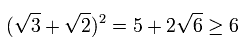And the D1 side can not detect the photon by canceling the waves.

(Eq.2) Light intensity at D1.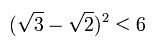As a result, the wave nature of Fig.2 can be explained using the classical electromagnetic waves naturally !
This means the photon is originally NOT a particle but an electromagnetic wave. (= "Photon particle" is a illusion. )
This is the trick of the delayed choice experiment, and unfortunately we can not change (choose) the past event from the future.

## Delayed choice quantum eraser can be explained by classical waves.

### Which-path information changes photon into a particle.

Using the concept of Wheeler's delayed choice experiment, the "delayed choice quantum eraser" experiment was performed (Phys. Rev. Lett. 2000 84 1-5).
In this delayed choice quantum eraser experiment, depending on the measument devices, the photon changes to particle or wave.

When we know which route the photon has passed, the photon changes to a particle !
But when we erase the information of which route the photon has passed, the superposition state of a single photon into the two paths is restored, and the photon changes to wave !
(= Delayed choice quantum eraser. )
But is this strange phenomenon really occurring ??

## Photon is wave or a particle ? ← we can control ?

(Fig.4) Delayed choice quantum eraser experiment.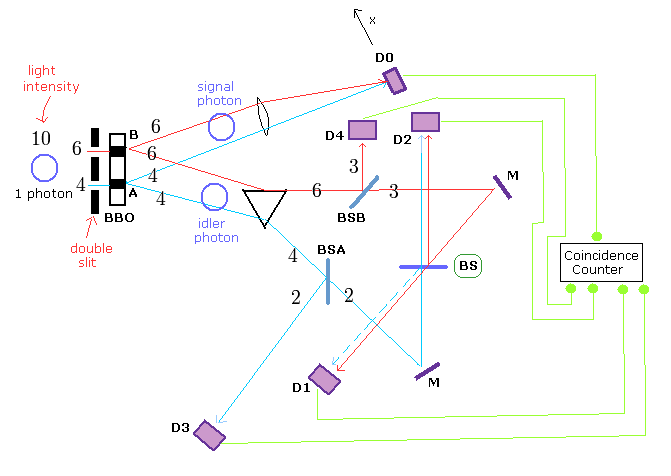In this experiment, an single photon is generated by Argon ion pump laser beam.
This laser (= single photon) is divided by the double slit into A and B paths (= what we call, "superposition" ).
( Or this single photon passes only one of slits, upper (= B ) or lower (= A ).)

After passing the slits, the beta barium crystal ( BBO ) causes spontaneous parametric down conversion (SPDC), converting the photon into two identical entangled photons with 1/2 the frequency of the original photon.
So the total energy is conserved.
( Surprisingly, a single photon particle can be divided into two particles by BBO ! )

In Fig.4 case, the light (= single photon ) with the intensity 10 is divided into 6+4 at the double slit apparatus.
And at each route ( A and B ), a pair of two identical photons with 1/2 frequency is generated ( 6 → 6+6, 4 → 4+4 ).

One of the two engtangled photons is sent to the upper route, and detected at D0 detector (= signal photon ).
( The position of the D0 detector can be moved in the x direction, as shown in Fig.4. )
And another photon (= idler photon ) is sent to the lower route, divided at the half beam splitters such as BSA, BSB, and BS.
( Of course, single photon particle can not be divided by the beam splitter according to the quantum mechanics.)

## When photon is detected at D3 or D4 → which-path information (= A or B ) is gotten ?

As shown in Fig.4, when we detect the idler photon at D4, we can know the information that the idler photon (= single photon ) has passed the "B" slit.
(= which-path information ).

When we detect the idler photon at D3, we can know that the idler photon has passed the "A" slit.
Suprisingly, in these cases ( D3 or D4 detection ), the signal photon at D0 shows the "particle" pattern of the double slit experiment !

At the last beam splitter (= "BS" of Fig.4), only one reflection is supposed to reverse the light phase, as shown in Fig.5.
( The transmitted light doesn't change. )
(Fig.5)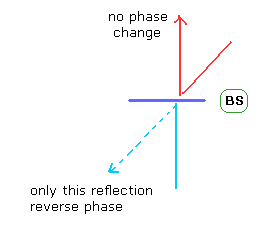So if the idler photon has the "wave" property, the light can not be detected at D1 detector, because their waves always cancel each other.
And when we detect the idler photon at D2 (of course, in this case D3 and D4 can't detect the idler photon ), we can not know the idler photon has passed A or B.
Because the light from both A and B routes can enter the D2 detector (and interfere with each other).

## When a photon is detected at D2, interference of a single photon happened !?

Suprisingly, in this case ( D2 detection ), the signal photon at D0 shows the "wave" interference pattern of the double slit experiment !
This means when we choose the detector ( D2, D3, or D4 ), the signal photon changes to particle or wave depending on it !

If we use D2 detector, the which-path information is erased, which means the superposition state of single photon is restored.
So the interference of the signal photon with itself occurs according to the quantum mechanical interpretation.
But does this strange phenomenon really occur ??

## " Trick " of delayed choice quantum eraser.

Here we explain the reason why D0 detector shows "particle" pattern of double slit experiment when we detect the idler photon at D3 or D4 detectors.
( Here we use only the classical electromagnetic wave theory to explain the delayed choice eraser like the upper section. )

(Fig.6) Which-path information ( A or B ) changes the photon into a "particle" ??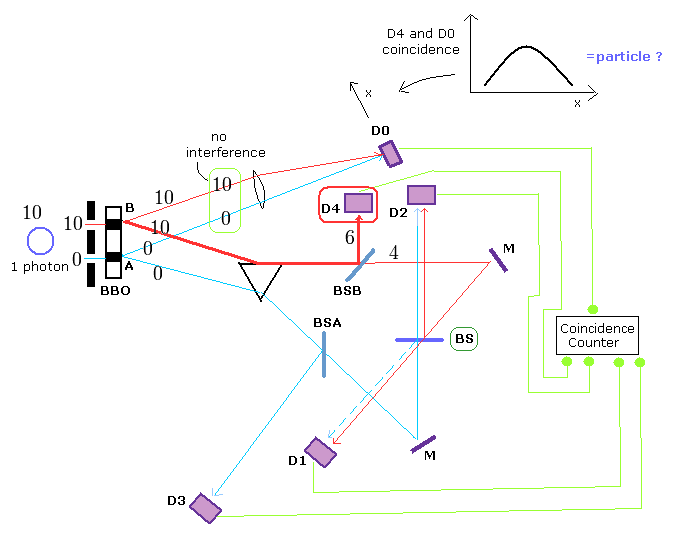As shown in Fig.6, when we detect the idler photon at D4 detector, it means the idler photon passes the "B" route ( NOT "A" ).
The first single photon intensity is 10, and almost all of the light needs to pass the upper slit (= "B" ) for us to detect it at D4.
Because at the first half beam splitter (= BSB ), the light is split into almost half ( in the classical waves ).
Like the upper section, the light intensity needs to reach at least "6" to detect it as a single particle.

## Detected at D4 → particle nature of photon appears ?

If the first light is divided at the double slit into A and B routes (for example, 10 → 4 (A) + 6 (B) ) like Fig.4, we can detect the idler photon at neither D3 nor D4.
Because at the first half beam splitter ( BSA or BSB ), the light is divided again ( for example, 6 → 3+3 ), which does NOT reach the threshold detection light intensity "6".

So, when we detect the idler photon at D4 (or D3 ), it means the first light passes ONLY "B" path (or only "A" path ).
As a result, the interference between A and B lights doesn't occur in the upward direction (= "D0" ), which shows "particle" pattern of Young double slit experiment.

## Detected at D2 → wave nature (= interference ) of photon ?

(Fig.7) "Erasing" which-path information ( A or B ) changes the photon into a "wave" ??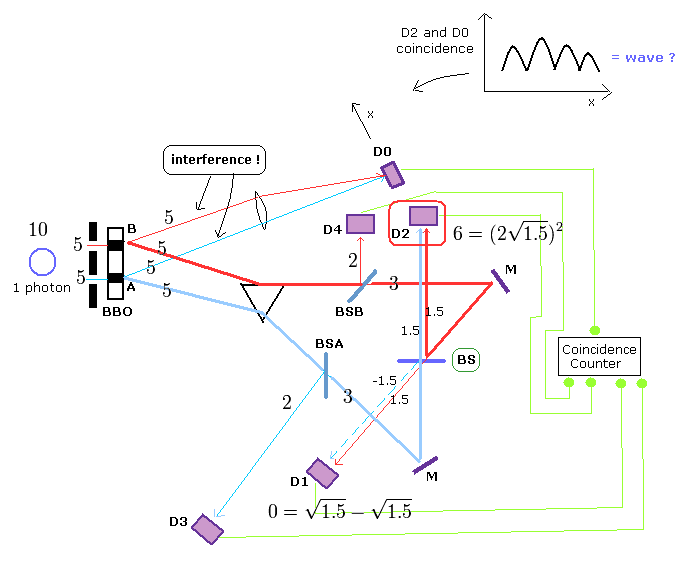Fig.7 shows the case in which we detect the idler photon at D2 detector.
Surplisingly, in these cases ( = D2 or D1 detection ), D0 detector shows the interference pattern between A and B lights.
Again, here we explain this trick using the usual classical electromagnetic waves.

If the first light passes only one ( A or B ) of the double slit as shown in Fig.6, can we detect the idler photon at D2 or D1 ?
Answer is NO ( we can detect the idler photon at neither D2 nor D1 when the first light is not divided at the double slit. ).
Because the light needs to pass two half beam splitters ( for example, BSB (BSA) and BS ) to reach D2 (or D1 ).
Then the first light intensity "10" becomes almost a quarter of the original value ( 10 → BSB (or BSA) → 5+5 → BS → 2.5 + 2.5 ) at D2 or D1, which is very difficult to detect as a photon ( we need "6" ).

To detect a single photon at D2 or D1, we have to take the power of the interference, as shown in Fig.7.
In Fig.7, the first light is divided into almost half "5+5" at the double slit.
After BBO, the idler photon ( = of course "classical" electromagnetic wave ) passes both the red and blue thick lines, and is divided again at BSB and BSA beam splitters ( for example, 5 → 3+2 ).
And furthermore, they are divided at the last beam splitter ( BS ) ( 3 → 1.5+1.5 ).
The light amplitude is a square root of the light intensity, and they are increased by the inteference from the red and blue lines.

## Classical lights + detection threshold is the trick !

(Eq.3) Light amplitude by the interference after BS.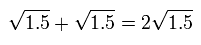The light intensity is a square of the light amplitude.
(Eq.4)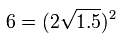As a result, we can detect the idler photon at D2 ONLY when the first light is divided into almost half at the double slit !
In this case ( Fig.7 ), the interference from A and B routes occur also at D0 detector, which shows "wave pattern".
This is a trick of the delayed choice quantum eraser.

As you notice, if we admit the particle nature of the photon, we have to accept the strange concepts such as entanglement, delayed-choice experiment, and many-world like superposition.
But if we treat the photon as usual classical electromagnetic wave, we need not accept those strange ideas.
You can easily judge which case ( photon is a particle or wave ) is more natural.2011/11/17 updated. Feel free to link to this site.